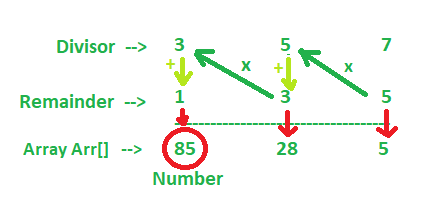# Find the number after successive division

Given two arrays div and rem which hold the values for divisors and remainders, the task is to find the number which after being successively divided by the elements of the div array leaves remainder which are in the rem array.

Note: The quotient from the first division will be divided by the second element and then the resultant quotient will be divided by the third (matching the remainders given) and so on.

Examples:

Input: div[] = {3, 5, 7}, rem[] = {1, 3, 5}
Output: 85
85 on division with 3 leaves remainder 1 with quotient 28
28 on division with 5 leaves remainder 3 with quotient 5
5 on division with 7 leaves remainder 5

Input: div[] = {7, 9}, rem[] = {2, 2}
Output: 16

## Recommended: Please try your approach on {IDE} first, before moving on to the solution.

Approach:• Store the value of the last remainder in a variable say num.
• Traverse the array backwards from n-2 to 0 and update the num as num = num * div[i] + rem[i]
• Print num in the end.

Below is the implementation of the above approach:

## C++

 `// C++ program to implement above approach ` `#include ` `using` `namespace` `std; ` ` `  `// Function to find the number ` `int` `findNum(``int` `div``[], ``int` `rem[], ``int` `N) ` `{ ` `    ``int` `num = rem[N - 1]; ` ` `  `    ``for` `(``int` `i = N - 2; i >= 0; i--) { ` `        ``num = num * ``div``[i] + rem[i]; ` `    ``} ` ` `  `    ``return` `num; ` `} ` ` `  `// Driver Code ` `int` `main() ` `{ ` `    ``int` `div``[] = { 8, 3 }; ` `    ``int` `rem[] = { 2, 2 }; ` `    ``int` `N = ``sizeof``(``div``) / ``sizeof``(``div``); ` ` `  `    ``cout << findNum(``div``, rem, N); ` ` `  `    ``return` `0; ` `} `

## Java

 `// Java implementation of the above approach ` ` `  `public` `class` `GFG{ ` `     `  `    ``// Function to find the number ` `    ``static` `int` `findNum(``int` `div[], ``int` `rem[], ``int` `N) ` `    ``{ ` `        ``int` `num = rem[N - ``1``]; ` `     `  `        ``for` `(``int` `i = N - ``2``; i >= ``0``; i--) { ` `            ``num = num * div[i] + rem[i]; ` `        ``} ` `     `  `        ``return` `num; ` `    ``} ` ` `  ` `  `     ``// Driver Code ` `     ``public` `static` `void` `main(String []args){ ` `            ``int` `div[] = { ``8``, ``3` `};  ` `            ``int` `rem[] = { ``2``, ``2` `};  ` `            ``int` `N = div.length;  ` `   `  `            ``System.out.println(findNum(div, rem, N));  ` ` `  `     ``} ` `     ``// This code is contributed by ANKITRAI1 ` `} `

## Python3

 `# Python 3 program to implement ` `# above approach ` ` `  `# Function to find the number ` `def` `findNum(div, rem, N): ` `    ``num ``=` `rem[N ``-` `1``] ` `    ``i ``=` `N ``-` `2` `    ``while``(i >``=` `0``): ` `        ``num ``=` `num ``*` `div[i] ``+` `rem[i] ` `         `  `        ``i ``-``=` `1` ` `  `    ``return` `num ` ` `  `# Driver Code ` `if` `__name__ ``=``=` `'__main__'``: ` `    ``div ``=` `[``8``, ``3``] ` `    ``rem ``=` `[``2``, ``2``] ` `    ``N ``=` `len``(div) ` ` `  `    ``print``(findNum(div, rem, N)) ` ` `  `# This code is contributed by ` `# Surendra_Gangwar `

## C#

 `// C# implementation of the  ` `// above approach  ` `using` `System; ` ` `  `class` `GFG ` `{ ` ` `  `// Function to find the number  ` `static` `int` `findNum(``int` `[]div, ` `                   ``int` `[]rem, ``int` `N)  ` `{  ` `    ``int` `num = rem[N - 1];  ` ` `  `    ``for` `(``int` `i = N - 2; i >= 0; i--) ` `    ``{  ` `        ``num = num * div[i] + rem[i];  ` `    ``}  ` ` `  `    ``return` `num;  ` `}  ` ` `  ` `  `// Driver Code  ` `static` `public` `void` `Main () ` `{ ` `    ``int` `[]div = { 8, 3 };  ` `    ``int` `[]rem = { 2, 2 };  ` `    ``int` `N = div.Length;  ` ` `  `    ``Console.WriteLine(findNum(div, rem, N));  ` ` `  `}  ` `}  ` ` `  `// This code is contributed by ajit `

## PHP

 `= 0; ``\$i``--) ` `    ``{ ` `        ``\$num` `= ``\$num` `* ``\$div``[``\$i``] + ``\$rem``[``\$i``]; ` `    ``} ` ` `  `    ``return` `\$num``; ` `} ` ` `  `// Driver Code ` `\$div` `= ``array``( 8, 3 ); ` `\$rem` `= ``array``(2, 2 ); ` `\$N` `= sizeof(``\$div``); ` ` `  `echo` `findNum(``\$div``, ``\$rem``, ``\$N``); ` ` `  `// This code is contributed by ajit ` `?> `

Output:

```18
```

My Personal Notes arrow_drop_upCheck out this Author's contributed articles.

If you like GeeksforGeeks and would like to contribute, you can also write an article using contribute.geeksforgeeks.org or mail your article to contribute@geeksforgeeks.org. See your article appearing on the GeeksforGeeks main page and help other Geeks.

Please Improve this article if you find anything incorrect by clicking on the "Improve Article" button below.